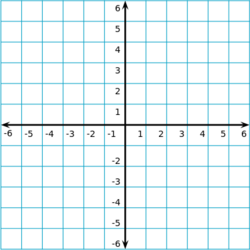## ASVAB Math Knowledge Practice Test 973276

Question 1 of 5
###### Slope-Intercept Equation

A line on the coordinate grid can be defined by a slope-intercept equation: y = mx + b. For a given value of x, the value of y can be determined given the slope (m) and y-intercept (b) of the line. The slope of a line is change in y over change in x, $${\Delta y \over \Delta x}$$, and the y-intercept is the y-coordinate where the line crosses the vertical y-axis.The endpoints of this line segment are at (-2, 4) and (2, 2). What is the slope of this line?

 -1 3 -$$\frac{1}{2}$$ -3